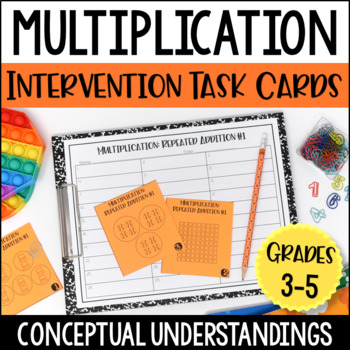# Multiplication Intervention Task Cards | Multiplication Conceptual Understanding3rd - 5th
Subjects
Resource Type
Formats Included
• PDF
Pages
50+

### Description

Students often struggle with multiplication fact fluency and automaticity because they lack a conceptual understanding of multiplication. This simple-to-prep and use multiplication intervention resource includes over 300 multiplication task cards to help you build conceptual understanding of multiplication with your students.

I created these conceptual multiplication task cards to be an extension of my multiplication intervention mini-lessons. For example, I will teach a small group lesson on representing multiplication with arrays and then the students will complete the task cards with me, as a math center, with partners, or independently.

This resource includes multiplication task cards for:

• Arrays
• Equal Group Models
• Strip Diagrams

***Click here to grab a free multiplication assessment to help you know which task cards to use with which students.

More Details About the Multiplication Intervention Task Cards

Here is a detailed list of the conceptual multiplication skills included. Each skill includes a task card set of 24 task cards:

• Writing the multiplication problem that matches the array and then solving.
• Using cubes or other small objects to create arrays, writing multiplication problem, and solving.
• Finishing array models to represent multiplication problems shown. Then solving the multiplication problems.
• Writing the multiplication problems that match the equal groups models and then solving the problems.
• Writing the multiplication problems that match the equal groups models and then solving the problems.
• Using cubes or other small objects to create equal group models, writing multiplication problems, and solving.
• Finishing equal groups models to represent the multiplication problems shown. Then solving the multiplication problems.
• Writing the multiplication problem that matches the strip diagram and then solving.
• Creating a strip diagram to model situations. Then writing multiplication problems and solving.
• Finishing strip diagram models to represent the multiplication problems shown. Then solving the multiplication problems.
• Writing repeated addition problems to match models. Optional version includes writing matching multiplication problems as well.
• Writing repeated addition problems and multiplication problems to match situations.
• Writing multiplication problems to match repeated addition problems.

This resource works well for students in grades 3-5 who have received initial instruction on multiplication but struggle to understand the skill conceptually.

Answer keys, direction pages, and recording sheets are included for each task card set.

Total Pages
50+
Included
Teaching Duration
1 month
Report this resource to TpT
Reported resources will be reviewed by our team. Report this resource to let us know if this resource violates TpT’s content guidelines.

### Questions & Answers

Teachers Pay Teachers is an online marketplace where teachers buy and sell original educational materials.